### 4.4.2 Characteristic Constraints

The constraints characteristic of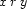or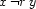for each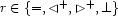can be easily expressed in terms of the node representations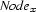and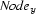.

proc {Equal N1 N2} N1=N2 end proc {NotEqual N1 N2}   {FS.disjoint N1.eq N2.eq}end proc {Above N1 N2}   {FS.subset N2.eqdown N1.down}   {FS.subset N1.equp N2.up}   {FS.subset N1.side N2.side}end proc {NotAbove N1 N2}   {FS.disjoint N1.eq N2.up}   {FS.disjoint N2.eq N1.down}end proc {Disjoint N1 N2}   {FS.subset N1.eqdown N2.side}   {FS.subset N2.eqdown N1.side}end proc {NotDisjoint N1 N2}   {FS.disjoint N1.eq N2.side}   {FS.disjoint N2.eq N1.side}end

Denys Duchier
Version 1.2.0 (20010221)